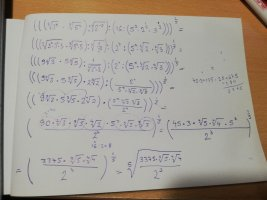# Help!

#### Loki123

##### Junior Member
This is the problem:This is how I tried to solve it:I failed badly. How do I do this?

#### topsquark

##### Senior Member
Okay, I don't know about you but the whole ratio symbol thing mixes me up. Anyway, the first thing I'd do would be to change [imath]16 = 2^4 = 2^{8}{2}[/imath]. Leave everything in terms of powers... don't multiply anything out. See if that helps.

-Dan

•Loki123

#### lev888

##### Elite Member
This is the problem:This is how I tried to solve it:
View attachment 29273
I failed badly. How do I do this?
2. Don't multiply, keep the original bases and only change exponents (see prev. post re 16).
3. Gradually get rid of the parentheses using the rules of exponents.
4. Change division to multiplication by flipping the sign of the denominator's exponent, e.g.: a/(b^(-2)) = ab^2.

•topsquark and Loki123

#### Loki123

##### Junior Member
Okay, I don't know about you but the whole ratio symbol thing mixes me up. Anyway, the first thing I'd do would be to change [imath]16 = 2^4 = 2^{8}{2}[/imath]. Leave everything in terms of powers... don't multiply anything out. See if that helps.

-Dan
What do you mean by this: 282 ?

#### Loki123

##### Junior Member
2. Don't multiply, keep the original bases and only change exponents (see prev. post re 16).
3. Gradually get rid of the parentheses using the rules of exponents.
4. Change division to multiplication by flipping the sign of the denominator's exponent, e.g.: a/(b^(-2)) = ab^2.
Yay! I solved it. It was surprisingly easy with those rules.

•lev888

#### topsquark

##### Senior Member
What do you mean by this: 282 ?
Honestly I don't know what I meant. I thought I had finished with [imath]16 = 2^4[/imath] !

-Dan[/imath]

•Loki123

#### Subhotosh Khan

##### Super Moderator
Staff member
Honestly I don't know what I meant. I thought I had finished with [imath]16 = 2^4[/imath] !

-Dan[/imath]
I think you tried to write:

$$\displaystyle 16 \ = \ \frac{2^5}{2}$$

•Loki123 and topsquark

#### topsquark

##### Senior Member
No. It was apparently a spasm of some kind. I have no clue.

-Dan

•Loki123